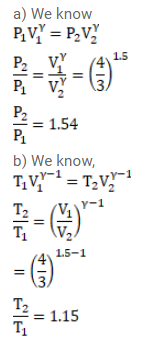# The volume of an ideal gas (???? = 1.5) is changed adiabaticallyQuestion:

The volume of an ideal gas $(\gamma=1.5)$ is changed adiabatically from $4.00$ liters to $3.00$ liters. Find the ratio of (a) the final pressure to the initial pressure and (b) the final temperature to the initial temperature.

Solution: